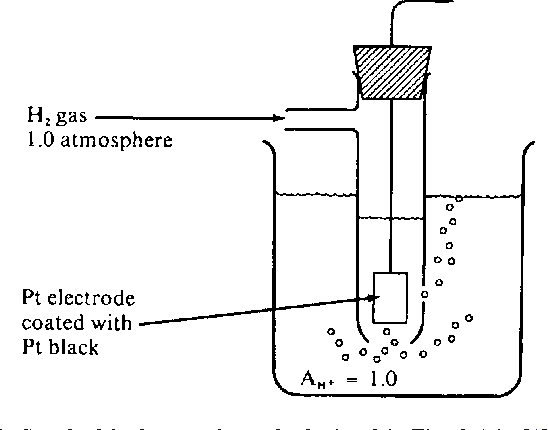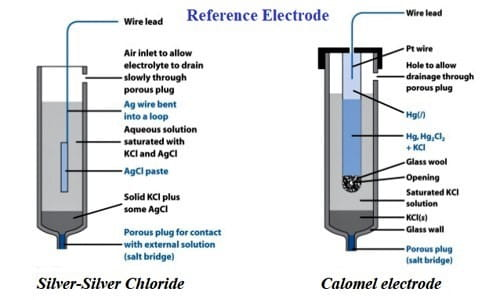# Some Typical Electrodes (or Half-Cells)

• A galvanic cell consists of two half-cells.
• In other words, a galvanic cell consists of two electrodes dipping into the same or two different electrolytes.
• There are several types of electrodes. Some commonly used electrodes are:

a) Metal-Metal ion electrode

• A metal-metal ion electrode consists of a metal rod or plate dipping into a solution containing its own ions.
• When such an electrode is used as a cathode, it is described as Mn+ (aq) | M(s), while if used as anode it is described as, M(s) | Mn+(aq).
• The electrode reactions for a metal-metal ion electrode may be written as,

Mn+ + ne(reduction/oxidation) ⇌   M(s)

• For example , when a silver wire is dipped into  a solution containing Ag+, the half cells are described as

Cathodic half-cell: Ag+(aq) | Ag

Anodic half-cell: Ag(s) | Ag+(aq)

The electrode reactions are

Ag+ + e(reduction/oxidation)   ⇌    Ag

b) Hydrogen Electrode

• Hydrogen electrode consists of hydrogen gas in equilibrium with H+ ions in a solution.
• Electrical contact of this electrode is provided by an inert metal like platinum.
• When this electrode is used as an anode, it is denoted by (Pt) H2(g) | H+(aq) and when this electrode is used as a cathode, it is denoted by H+(aq) | H2(g)(Pt)
• The electrode reactions are,

H+(aq) + e(reduction/oxidation) ⇌ ½ H2(g)

The emf of the hydrogen electrode depends upon, a) concentration of H+ ions in solution  b) pressure of the hydrogen gas.

• A hydrogen electrode is obtained by bubbling hydrogen gas on platinum foil dipping into a solution containing H+.
• Generally, the platinum foil used in hydrogen electrode is coated with platinum black.Standard hydrogen electrode

A hydrogen electrode in which the pressure of hydrogen gas is maintained at 1 atm, and the concentration of H+ ions in the solution is 1M, is called a standard hydrogen electrode (SHE).

The emf of a standard hydrogen electrode is taken as 0.0V at all temperatures. That is the reason why standard hydrogen electrode is used as a primary reference electrode for measuring the emf of all other electrodes.

c) Calomel Electrode

• Most commonly used electrode is the calomel electrode.
• It consists of mercury in contact with calomel, (Hg2Cl2).
• A solution containing chlorine ions (e.g., a solution of KCl) is poured-over.
• A general set up for a calomel electrode is shown in the figure. Calomel electrode is written as:When it acts as a cathode: Cl(aq) | Hg2Cl2(s) | Hg(l)

When it acts as an anode: Hg(l) | Hg2Cl2(s) | Cl(aq)

Electrode reactions for the calomel electrode are,

½ Hg2Cl2(s) + e (reduction/oxidation) ⇌ Hg(l) + Cl(aq)

• Generally, 0.1M, 1M, or a saturated solution of KCL is used in a calomel electrode.
• When the solution used in a calomel electrode is a saturated solution of KCl, then, the calomel electrode is called a saturated calomel electrode (SCE).
• The emf of the calomel electrode depends upon the concentration of Clions in the solution.
• Calomel electrode is commonly used as a secondary reference electrode, because its potential remains constant over a long period of time.

References: